Courses

# Test: Analysis of Stress & Strain - 1

## 10 Questions MCQ Test Strength of Materials (SOM) | Test: Analysis of Stress & Strain - 1

Description
This mock test of Test: Analysis of Stress & Strain - 1 for Mechanical Engineering helps you for every Mechanical Engineering entrance exam. This contains 10 Multiple Choice Questions for Mechanical Engineering Test: Analysis of Stress & Strain - 1 (mcq) to study with solutions a complete question bank. The solved questions answers in this Test: Analysis of Stress & Strain - 1 quiz give you a good mix of easy questions and tough questions. Mechanical Engineering students definitely take this Test: Analysis of Stress & Strain - 1 exercise for a better result in the exam. You can find other Test: Analysis of Stress & Strain - 1 extra questions, long questions & short questions for Mechanical Engineering on EduRev as well by searching above.
QUESTION: 1

### A bar of square cross - section has b ee n subjected to an axial tensile load. A plane normal to the axis of loading will have

Solution: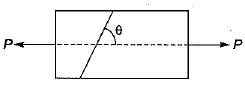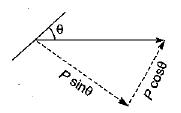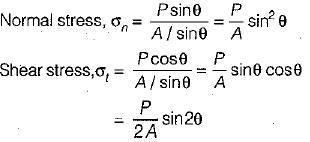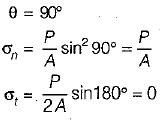QUESTION: 2

### Normal stresses of equal magnitude a but of opposite signs act at a point of a strained material in perpendicular direction. What would be the resultant normal stress on a plane inclined at 45° to the applied stresses?

Solution: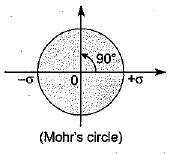QUESTION: 3

### With reference to the following figure, the tangential stress σt on a plane inclined at an angle θ to the line of action of σ1 would be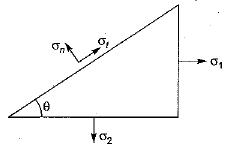Solution:
QUESTION: 4

For an element under the effect of biaxial state of normal stresses, the normal stress on 45° plane is equal to

Solution: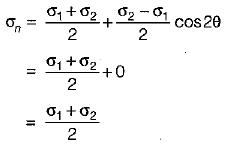QUESTION: 5

If the principal stresses on a plane stress problem are σ1 = 100 MPa and σ2 = 40 MPa, then the magnitude of shear stress (in MPa) will be

Solution: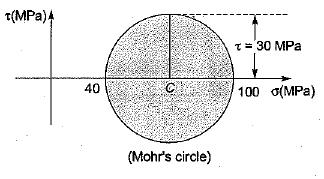QUESTION: 6

The state of plane stress at a point is given by σx = 200 MPa, σy = 100 MPa and τxy = 100 MPa. The maximum shear stress (in MPa) is then

Solution: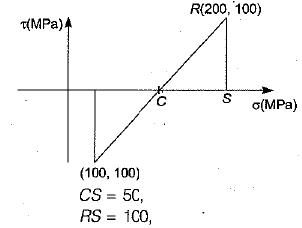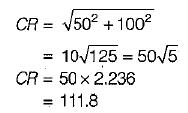Maximum shear stress = 111.8 MPa

QUESTION: 7

Identify the WRONG statement:

Solution:

Two principal planes carrying the maximum and direct stresses are always perpendicular to each other.

QUESTION: 8

Complimentary shear stresses are

Solution:
QUESTION: 9

The state of stresses on an element is as shown in following figure.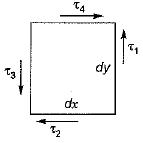The necessary and sufficient condition for its equilibrium is

Solution: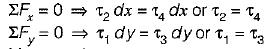Moment about upper right corner = 0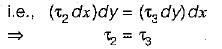Moment about lower left corner = 0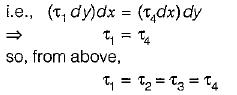QUESTION: 10

At the principal planes

Solution:

Principal stresses are maximum or minimum normal stresses which may occur on a stressed body. In a 3-D body there may three principal planes which are mutually perpendicular to each other. The plane of principle stresses is called principal plane which always carries zero shear stress.